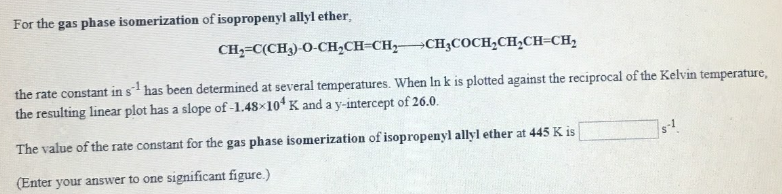# For the gas phase isomerization of isopropenyl allyl ether CH2=C(CH3)-O-CH2CH=CH2 → CH3COCH2CHCH=CH2 the rate constant in s^-1 has been determined at several temperatures. When ln k is plotted against the reciprocal of the Kelvin temperature, the resulting linear plot has a slope of -1.48x10^-4 and a y-intercept of 26.0. The value of the rate constant for the gas phase isomerization of isopropenyl allyl ether at 445 K is ____ s^-1. (Enter your answer to one significant figure.)